# NCERT Exemplar Solutions for Class 9 Maths Chapter 4 - Linear Equations In Two Variables

## NCERT Exemplar Solutions Class 9 Maths Chapter 4 – Free PDF Download

NCERT Exemplar Class 9 Maths Chapter 4 Linear Equations in Two Variables, is provided here in PDF format for students to prepare for exams. Our experts have designed exemplar problems with solutions in accordance with the CBSE syllabus for the 9th standard. The linear equation in two variables is one of the most important Chapters of Class 9. Questions of this chapter are also asked in competitive exams like CAT, IAS, and UPSC. Learning linear equations chapter using the NCERT exemplar will also help the students to score good marks in the examination. NCERT Exemplar Class 9 Maths Chapter 4 covers the topics which are given below:

• Problems based on linear equations in two variables
• Finding solutions to linear equations
• Representation of linear equations in two variables, graphically
• Linear equations of lines parallel to x-axis and y-axis in a graph

To encourage easy learning and help students understand the concepts of linear equations, free NCERT Exemplars are provided here which can be further downloaded in the form of a PDF. NCERT Exemplar Class 9 Maths solutions can be used as a reference tool by 9th standard students while practising the NCERT book exercise questions.

## Download PDF of NCERT Exemplar Solutions for Class 9 Maths Chapter 4 Linear Equations in Two Variables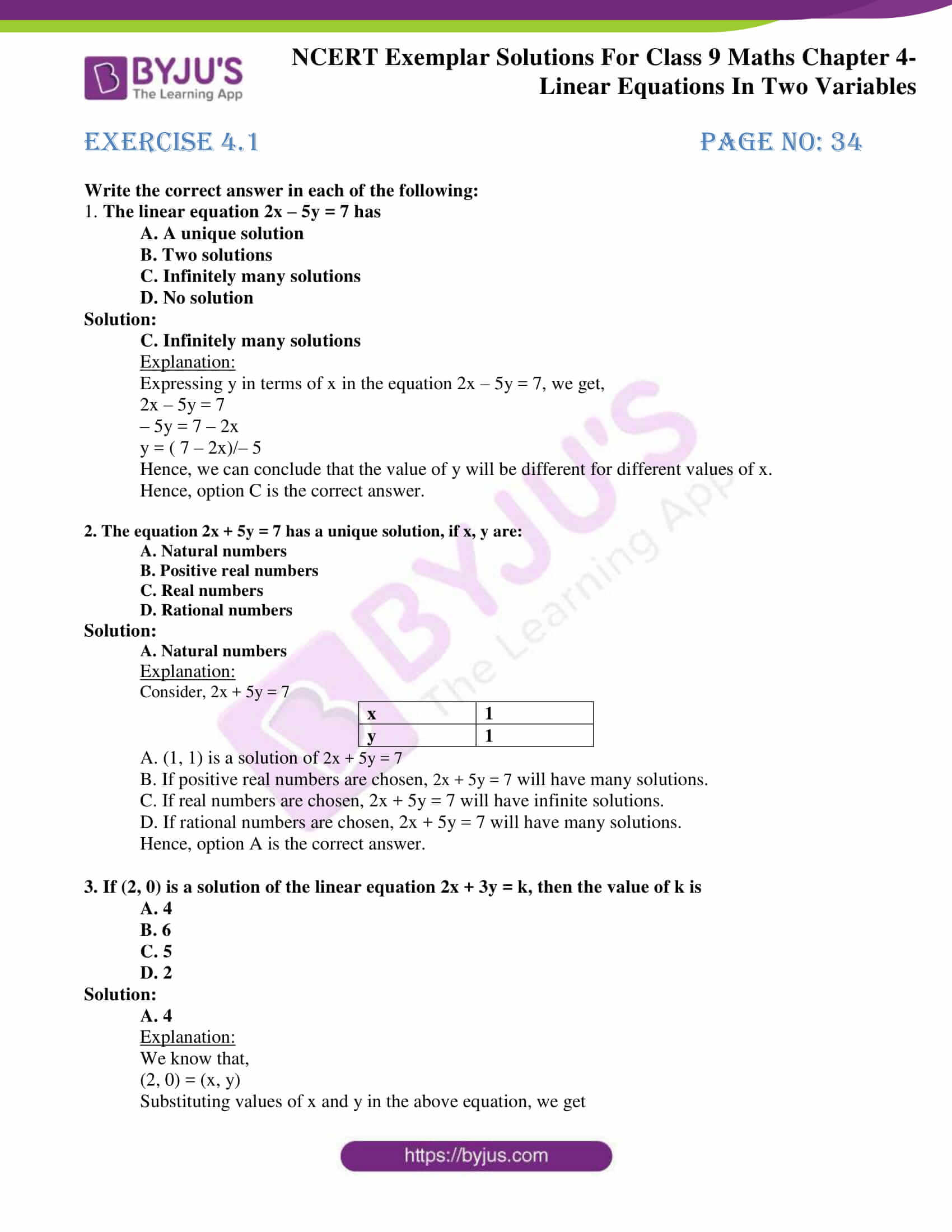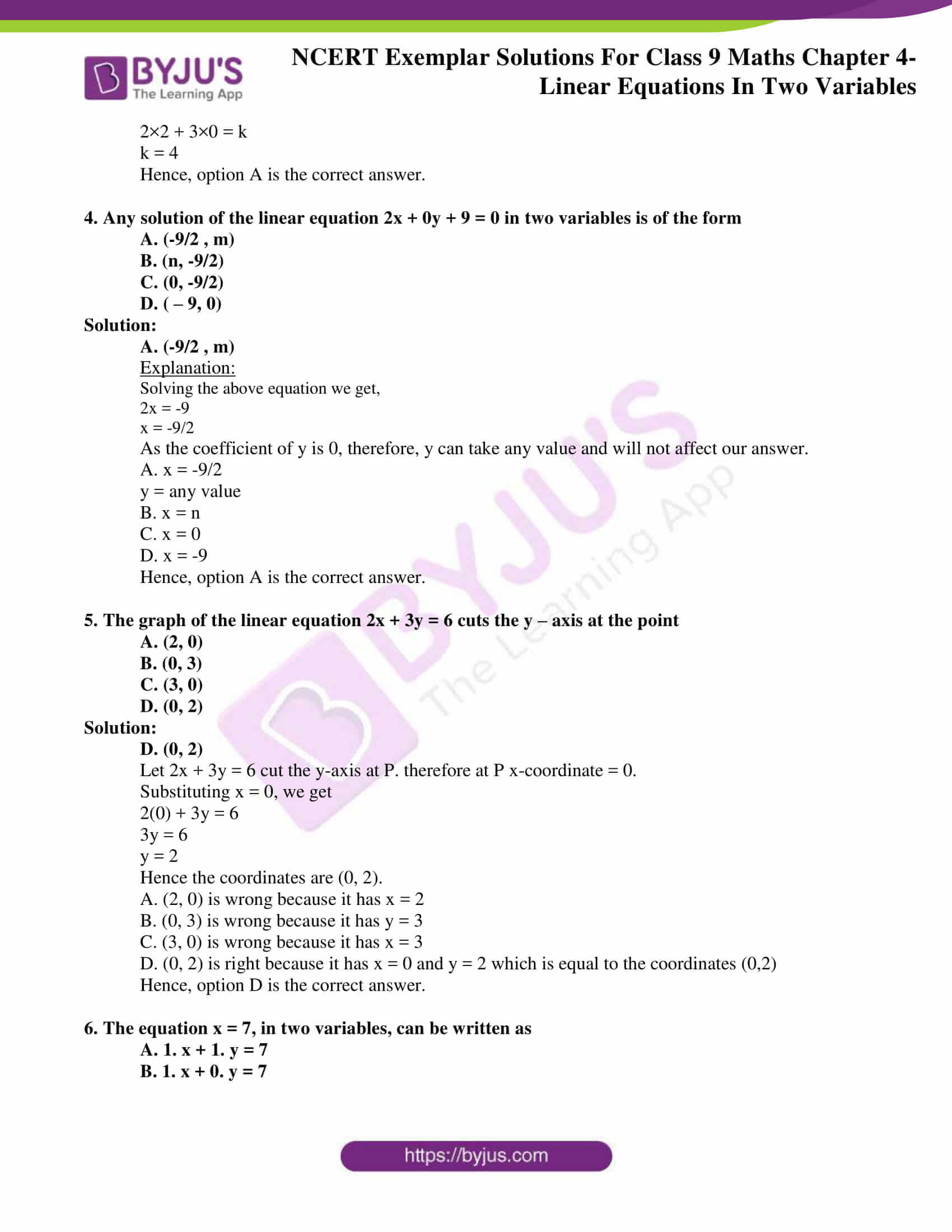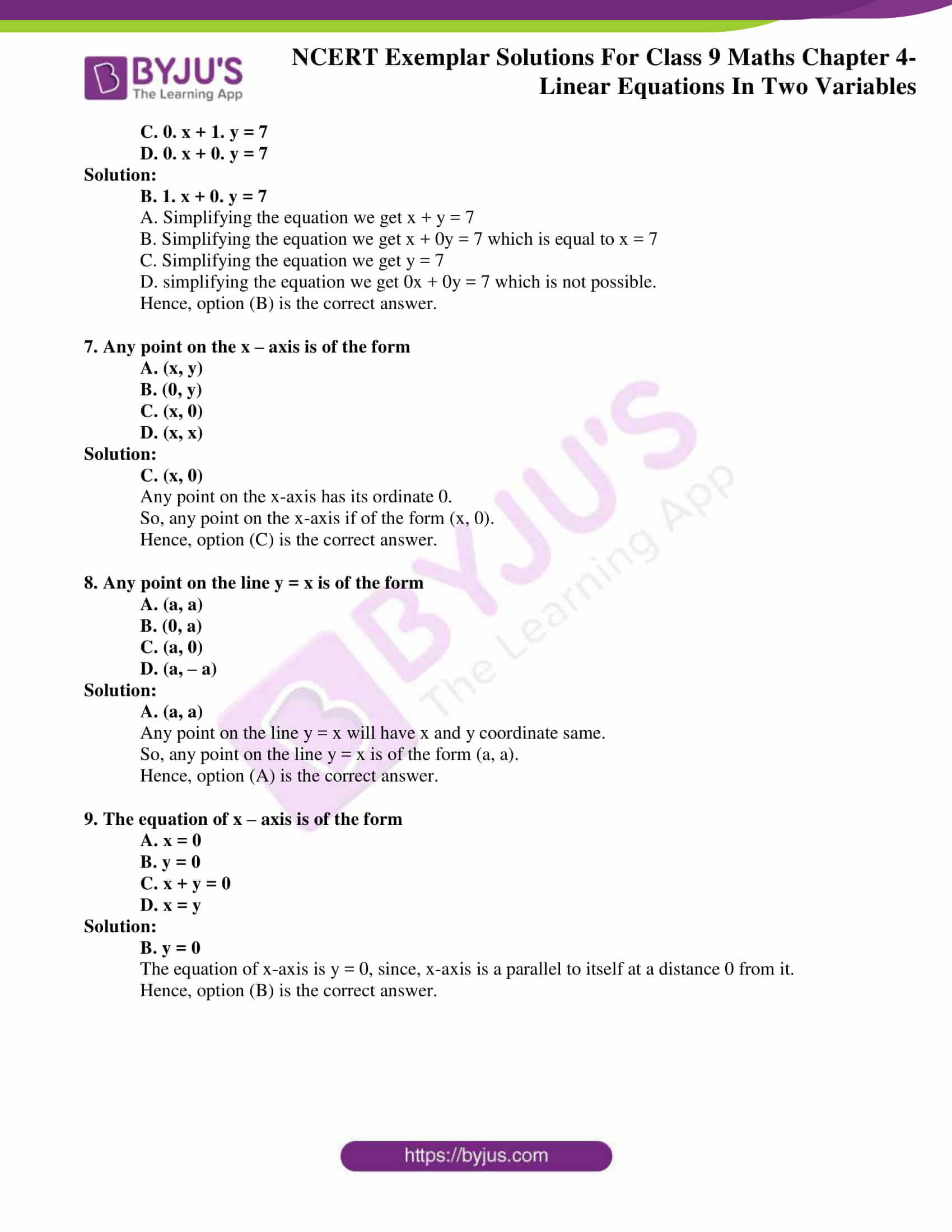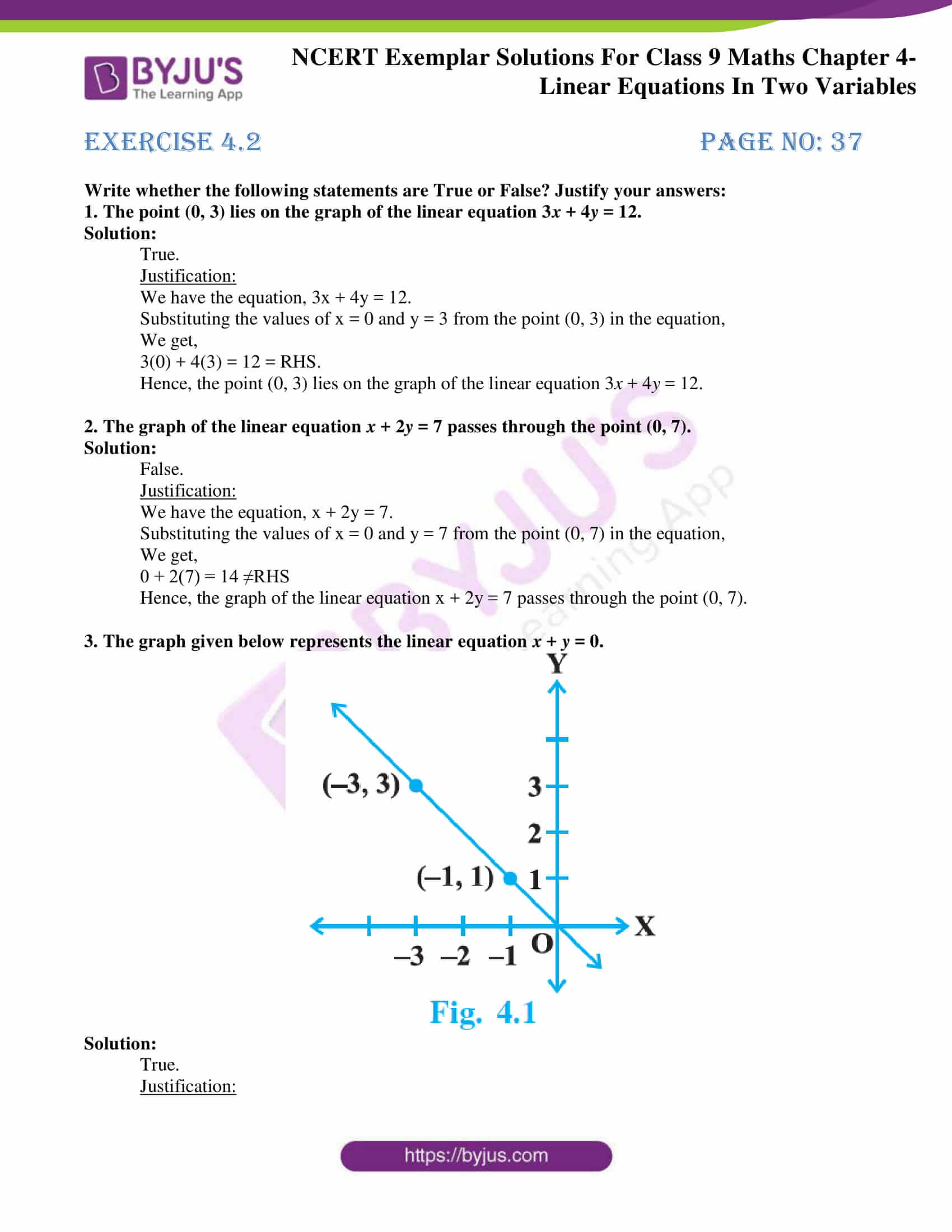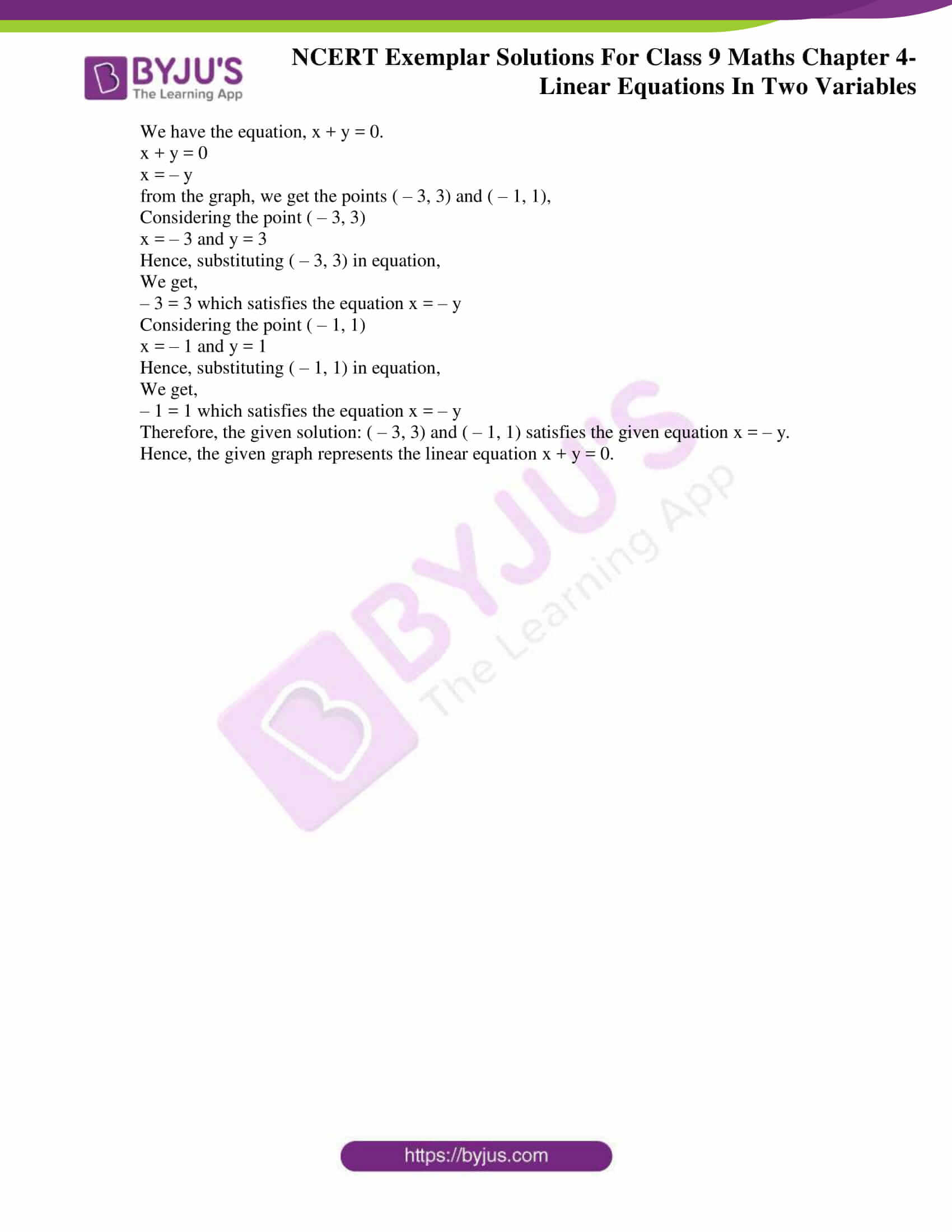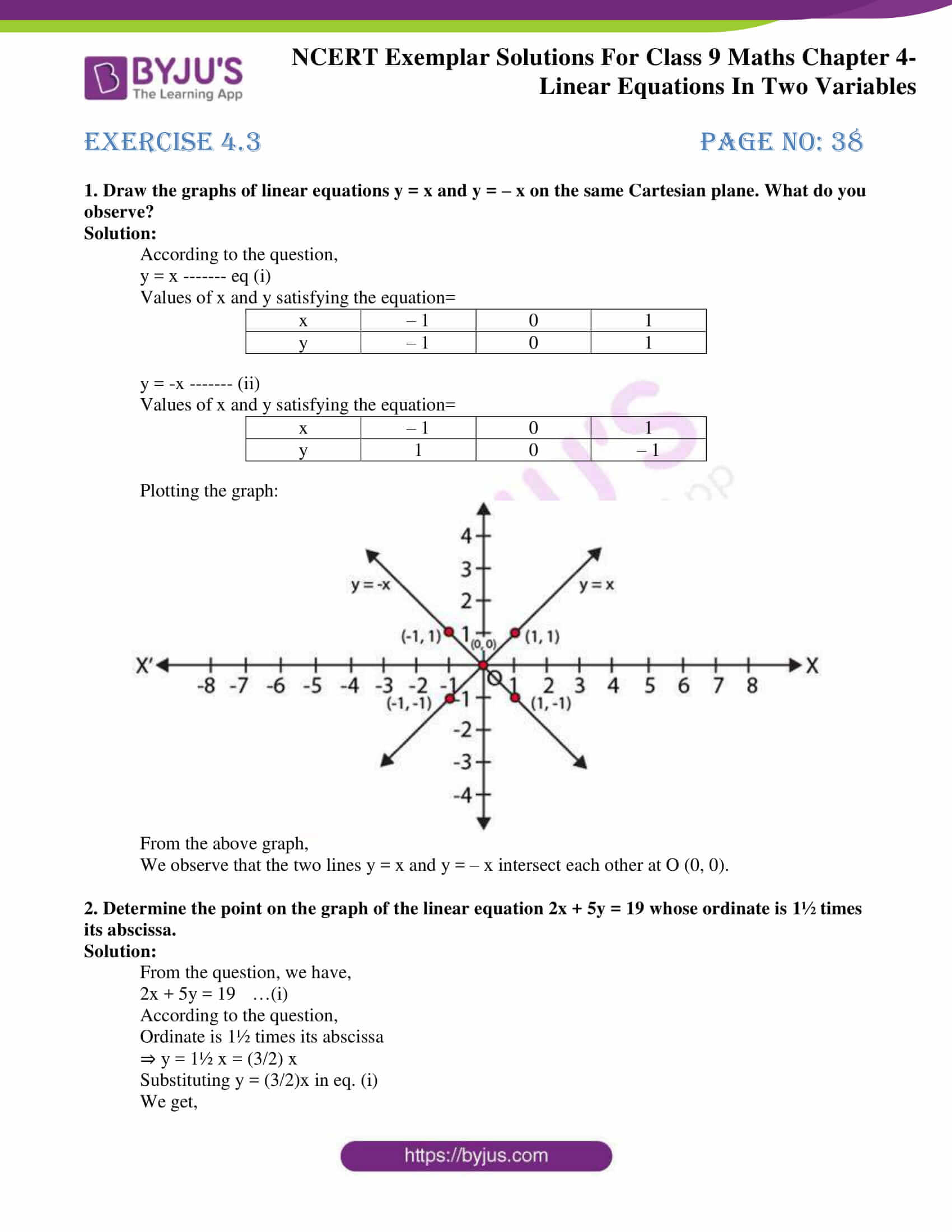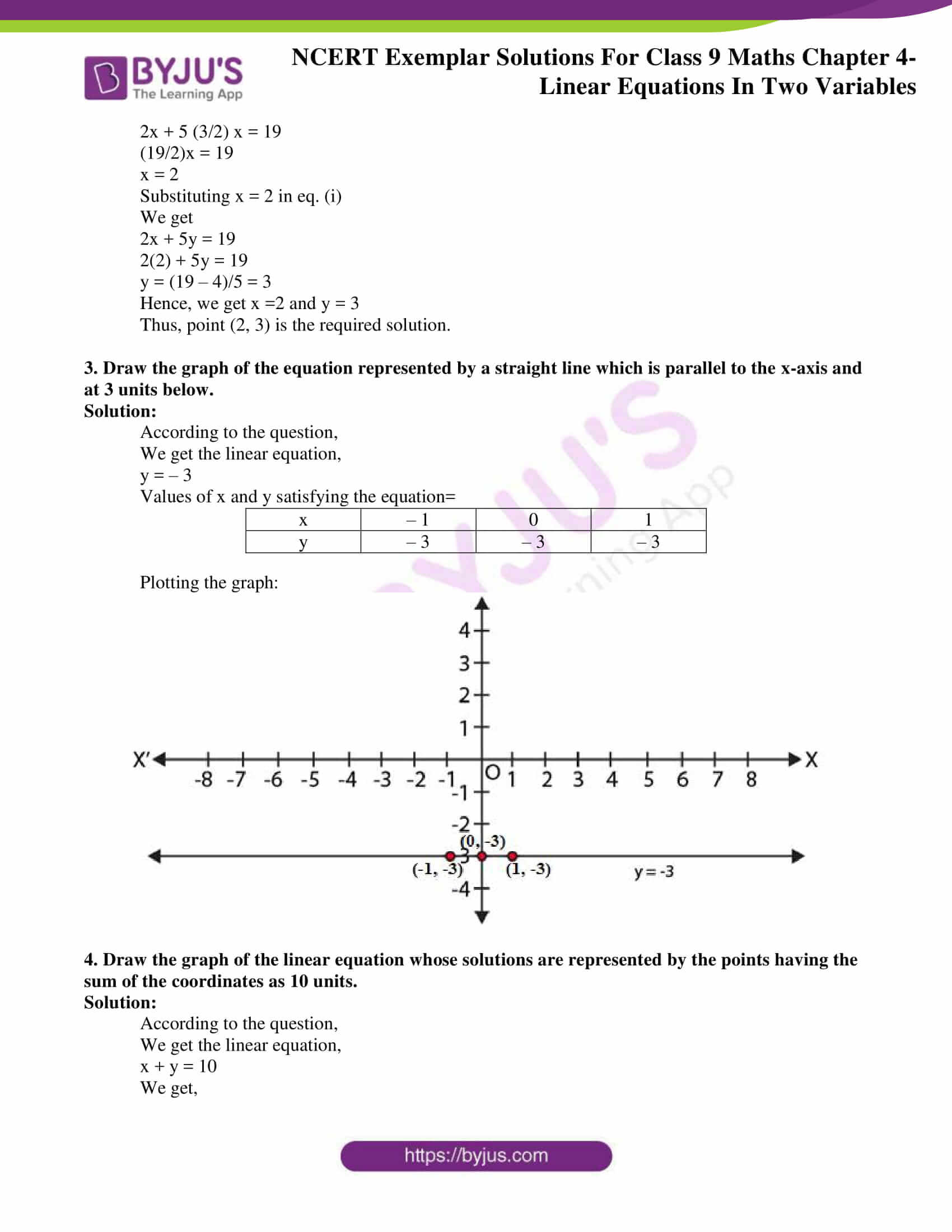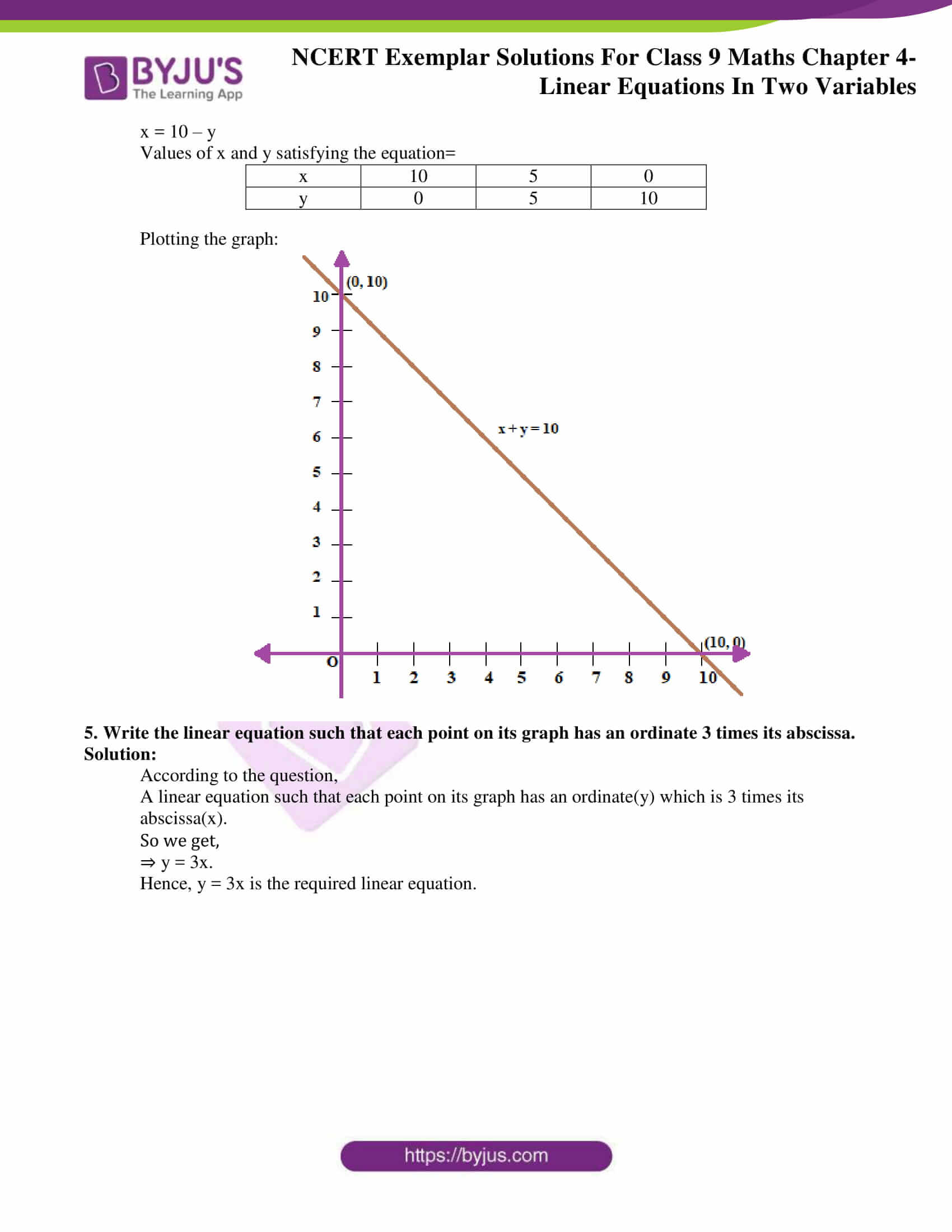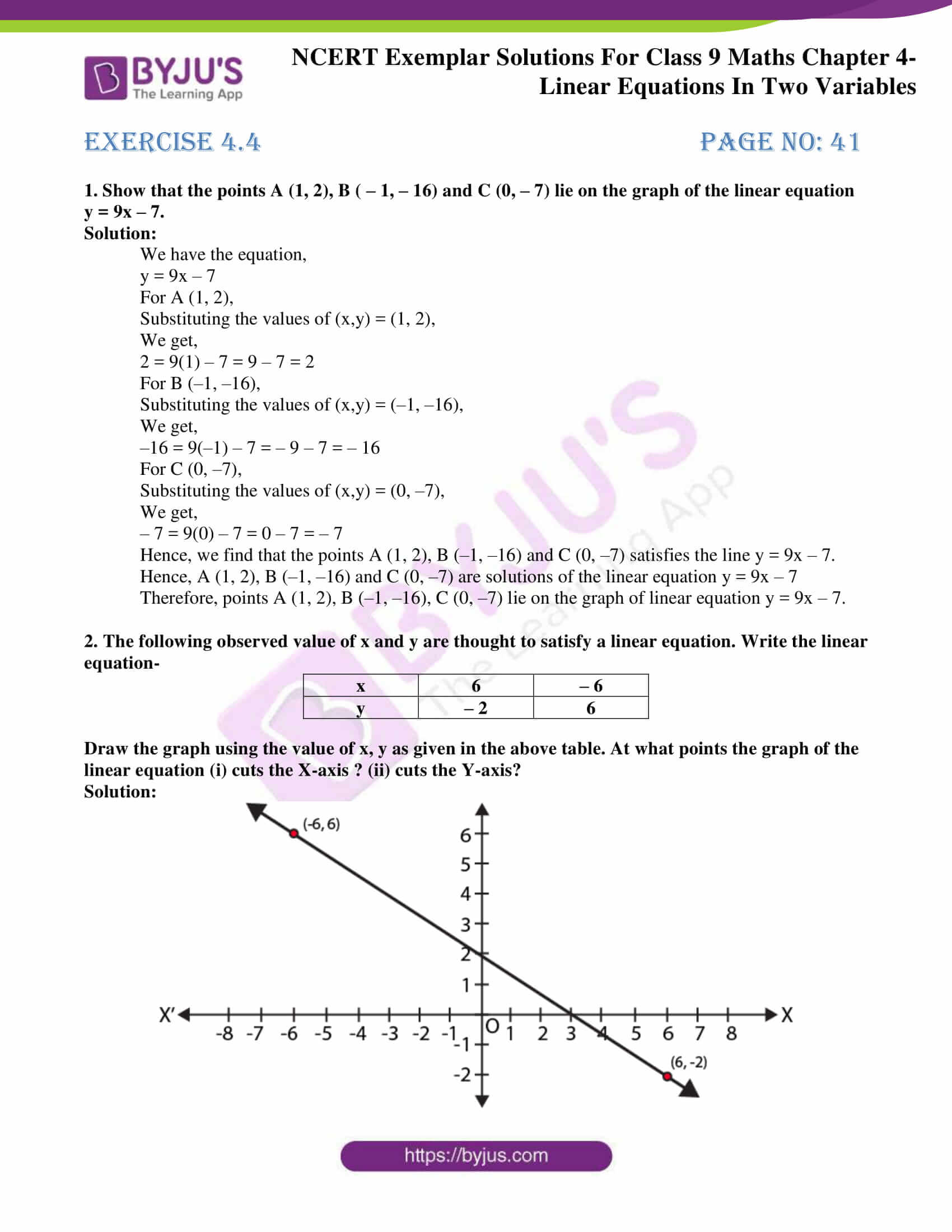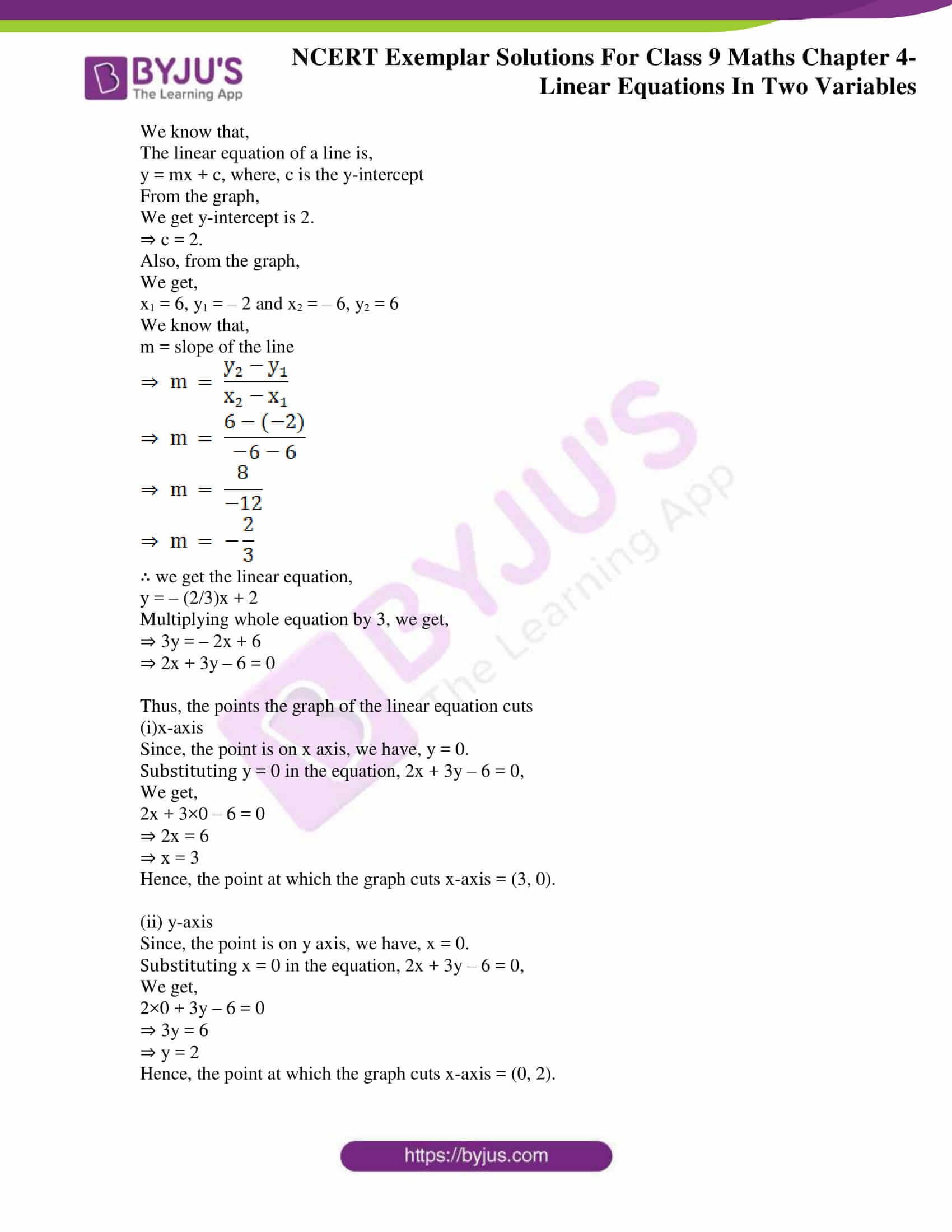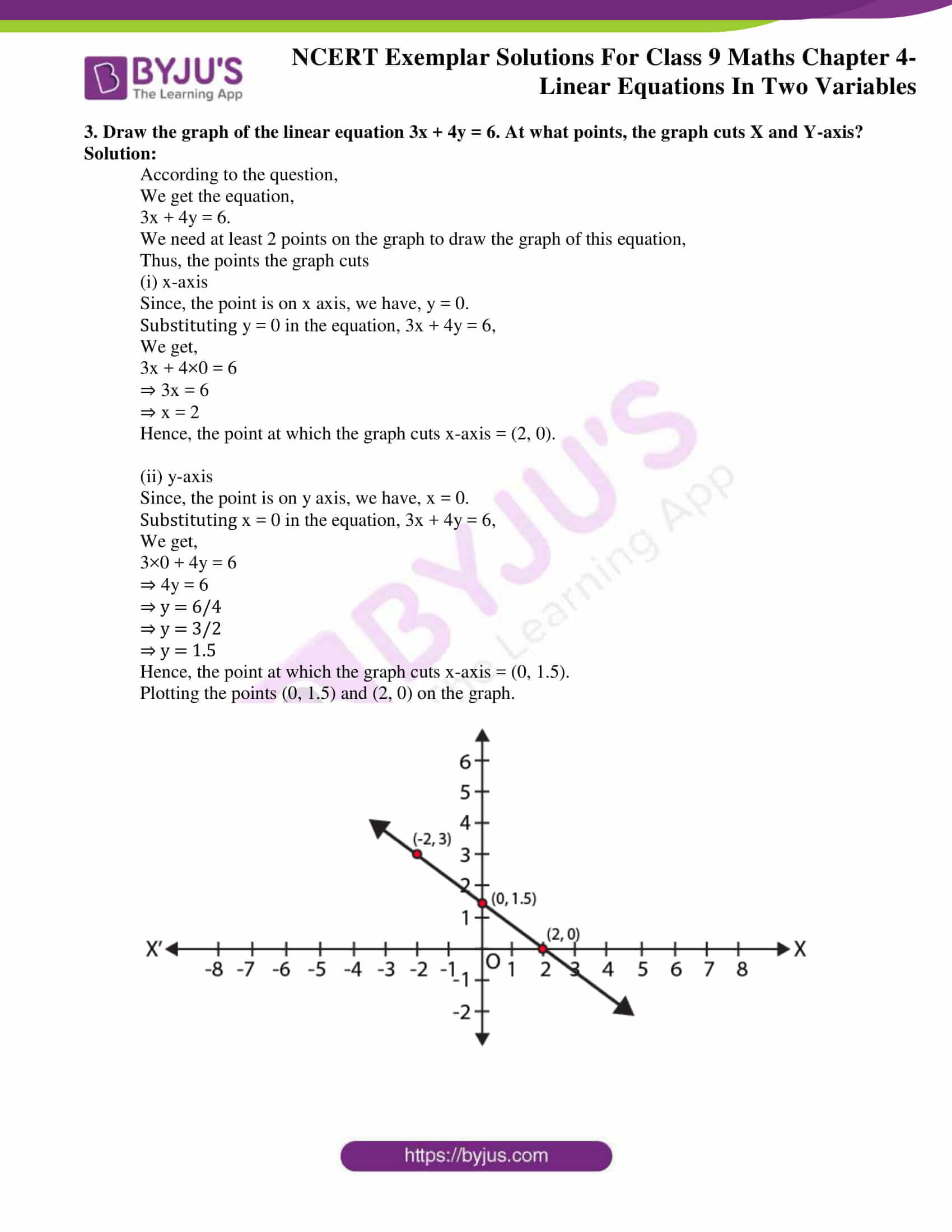### Access answers to NCERT Exemplar Solutions for Class 9 Maths Chapter 4 Linear Equations in Two Variables

Exercise 4.1 Page No: 34

Write the correct answer in each of the following:

1. The linear equation 2x – 5y = 7 has

A. A unique solution
B. Two solutions
C. Infinitely many solutions
D. No solution

Solution:

C. Infinitely many solutions

Explanation:

Expressing y in terms of x in the equation 2x – 5y = 7, we get,

2x – 5y = 7

– 5y = 7 – 2x

y = ( 7 – 2x)/– 5

Hence, we can conclude that the value of y will be different for different values of x.

Hence, option C is the correct answer.

2. The equation 2x + 5y = 7 has a unique solution, if x, y are:

A. Natural numbers

B. Positive real numbers

C. Real numbers

D. Rational numbers

Solution:

A. Natural numbers

Explanation:

Consider, 2x + 5y = 7

 x 1 y 1

A. (1, 1) is a solution of 2x + 5y = 7

B. If positive real numbers are chosen, 2x + 5y = 7 will have many solutions.

C. If real numbers are chosen, 2x + 5y = 7 will have infinite solutions.

D. If rational numbers are chosen, 2x + 5y = 7 will have many solutions.

Hence, option A is the correct answer.

3. If (2, 0) is a solution of the linear equation 2x + 3y = k, then the value of k is
A. 4
B. 6
C. 5
D. 2

Solution:

A. 4

Explanation:

We know that,

(2, 0) = (x, y)

Substituting values of x and y in the above equation, we get

2×2 + 3×0 = k

k = 4

Hence, option A is the correct answer.

4. Any solution of the linear equation 2x + 0y + 9 = 0 in two variables is of the form

A. (-9/2 , m)
B. (n, -9/2)
C. (0, -9/2)
D. ( – 9, 0)

Solution:

A. (-9/2 , m)

Explanation:

Solving the above equation we get,

2x = -9

x = -9/2

As the coefficient of y is 0, therefore, y can take any value and will not affect our answer.

A. x = -9/2

y = any value

B. x = n

C. x = 0

D. x = -9

Hence, option A is the correct answer.

5. The graph of the linear equation 2x + 3y = 6 cuts the y – axis at the point

A. (2, 0)
B. (0, 3)
C. (3, 0)
D. (0, 2)

Solution:

D. (0, 2)

Let 2x + 3y = 6 cut the y-axis at P. therefore at P x-coordinate = 0.

Substituting x = 0, we get

2(0) + 3y = 6

3y = 6

y = 2

Hence the coordinates are (0, 2).

A. (2, 0) is wrong because it has x = 2

B. (0, 3) is wrong because it has y = 3

C. (3, 0) is wrong because it has x = 3

D. (0, 2) is right because it has x = 0 and y = 2 which is equal to the coordinates (0,2)

Hence, option D is the correct answer.

6. The equation x = 7, in two variables, can be written as

A. 1. x + 1. y = 7
B. 1. x + 0. y = 7
C. 0. x + 1. y = 7
D. 0. x + 0. y = 7

Solution:

B. 1. x + 0. y = 7

A. Simplifying the equation we get x + y = 7

B. Simplifying the equation we get x + 0y = 7 which is equal to x = 7

C. Simplifying the equation we get y = 7

D. simplifying the equation we get 0x + 0y = 7 which is not possible.

Hence, option (B) is the correct answer.

7. Any point on the x – axis is of the form

A. (x, y)
B. (0, y)
C. (x, 0)
D. (x, x)

Solution:

C. (x, 0)

Any point on the x-axis has its ordinate 0.

So, any point on the x-axis if of the form (x, 0).

Hence, option (C) is the correct answer.

8. Any point on the line y = x is of the form

A. (a, a)
B. (0, a)
C. (a, 0)
D. (a, – a)

Solution:

A. (a, a)

Any point on the line y = x will have x and y coordinate same.

So, any point on the line y = x is of the form (a, a).

Hence, option (A) is the correct answer.

9. The equation of x – axis is of the form

A. x = 0
B. y = 0
C. x + y = 0
D. x = y

Solution:

B. y = 0

The equation of x-axis is y = 0, since, x-axis is a parallel to itself at a distance 0 from it.

Hence, option (B) is the correct answer.

Exercise 4.2 Page No: 37

Write whether the following statements are True or False? Justify your answers:

1. The point (0, 3) lies on the graph of the linear equation 3x + 4y = 12.

Solution:

True.

Justification:

We have the equation, 3x + 4y = 12.

Substituting the values of x = 0 and y = 3 from the point (0, 3) in the equation,

We get,

3(0) + 4(3) = 12 = RHS.

Hence, the point (0, 3) lies on the graph of the linear equation 3x + 4y = 12.

2. The graph of the linear equation x + 2y = 7 passes through the point (0, 7).

Solution:

False.

Justification:

We have the equation, x + 2y = 7.

Substituting the values of x = 0 and y = 7 from the point (0, 7) in the equation,

We get,

0 + 2(7) = 14 ≠RHS

Hence, the graph of the linear equation x + 2y = 7 passes through the point (0, 7).

3. The graph given below represents the linear equation x + y = 0.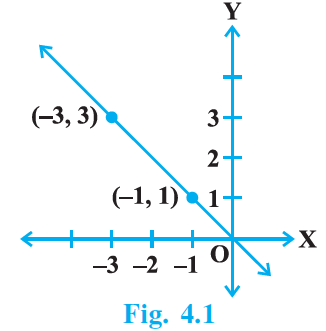Solution:

True.

Justification:

We have the equation, x + y = 0.

x + y = 0

x = – y

from the graph, we get the points ( – 3, 3) and ( – 1, 1),

Considering the point ( – 3, 3)

x = – 3 and y = 3

Hence, substituting ( – 3, 3) in equation,

We get,

– 3 = 3 which satisfies the equation x = – y

Considering the point ( – 1, 1)

x = – 1 and y = 1

Hence, substituting ( – 1, 1) in equation,

We get,

– 1 = 1 which satisfies the equation x = – y

Therefore, the given solution: ( – 3, 3) and ( – 1, 1) satisfies the given equation x = – y.

Hence, the given graph represents the linear equation x + y = 0.

Exercise 4.3 Page No: 38

1. Draw the graphs of linear equations y = x and y = – x on the same Cartesian plane. What do you observe?

Solution:

According to the question,

y = x ——- eq (i)

Values of x and y satisfying the equation=

 x – 1 0 1 y – 1 0 1

y = -x ——- (ii)

Values of x and y satisfying the equation=

 x – 1 0 1 y 1 0 – 1

Plotting the graph: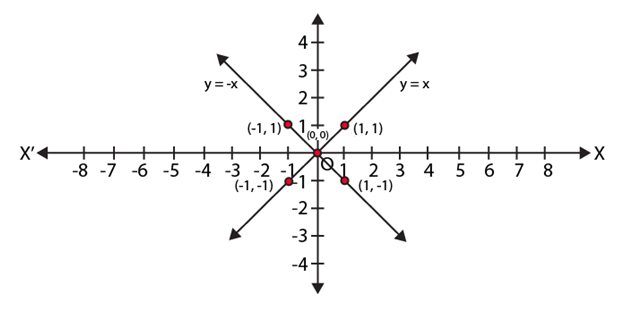From the above graph,

We observe that the two lines y = x and y = – x intersect each other at O (0, 0).

2. Determine the point on the graph of the linear equation 2x + 5y = 19 whose ordinate is 1½ times its abscissa.

Solution:

From the question, we have,

2x + 5y = 19 …(i)

According to the question,

Ordinate is 1½ times its abscissa

⇒ y = 1½ x = (3/2) x

Substituting y = (3/2)x in eq. (i)

We get,

2x + 5 (3/2) x = 19

(19/2)x = 19

x = 2

Substituting x = 2 in eq. (i)

We get

2x + 5y = 19

2(2) + 5y = 19

y = (19 – 4)/5 = 3

Hence, we get x =2 and y = 3

Thus, point (2, 3) is the required solution.

3. Draw the graph of the equation represented by a straight line which is parallel to the x-axis and at 3 units below.

Solution:

According to the question,

We get the linear equation,

y = – 3

Values of x and y satisfying the equation=

 x – 1 0 1 y – 3 – 3 – 3

Plotting the graph: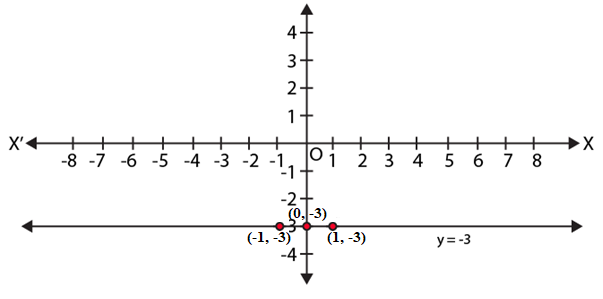4. Draw the graph of the linear equation whose solutions are represented by the points having the sum of the coordinates as 10 units.

Solution:

According to the question,

We get the linear equation,

x + y = 10

We get,

x = 10 – y

Values of x and y satisfying the equation=

 x 10 5 0 y 0 5 10

Plotting the graph: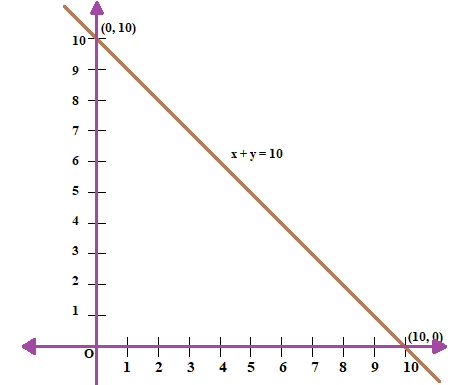5. Write the linear equation such that each point on its graph has an ordinate 3 times its abscissa.

Solution:

According to the question,

A linear equation such that each point on its graph has an ordinate(y) which is 3 times its abscissa(x).

So we get,

⇒ y = 3x.

Hence, y = 3x is the required linear equation.

Exercise 4.4 Page No: 41

1. Show that the points A (1, 2), B ( – 1, – 16) and C (0, – 7) lie on the graph of the linear equation y = 9x – 7.

Solution:

We have the equation,

y = 9x – 7

For A (1, 2),

Substituting the values of (x,y) = (1, 2),

We get,

2 = 9(1) – 7 = 9 – 7 = 2

For B (–1, –16),

Substituting the values of (x,y) = (–1, –16),

We get,

–16 = 9(–1) – 7 = – 9 – 7 = – 16

For C (0, –7),

Substituting the values of (x,y) = (0, –7),

We get,

– 7 = 9(0) – 7 = 0 – 7 = – 7

Hence, we find that the points A (1, 2), B (–1, –16) and C (0, –7) satisfies the line y = 9x – 7.

Hence, A (1, 2), B (–1, –16) and C (0, –7) are solutions of the linear equation y = 9x – 7

Therefore, points A (1, 2), B (–1, –16), C (0, –7) lie on the graph of linear equation y = 9x – 7.

2. The following observed value of x and y are thought to satisfy a linear equation. Write the linear equation-

 x 6 – 6 y – 2 6

Draw the graph using the value of x, y as given in the above table. At what points the graph of the linear equation (i) cuts the X-axis ? (ii) cuts the Y-axis?

Solution: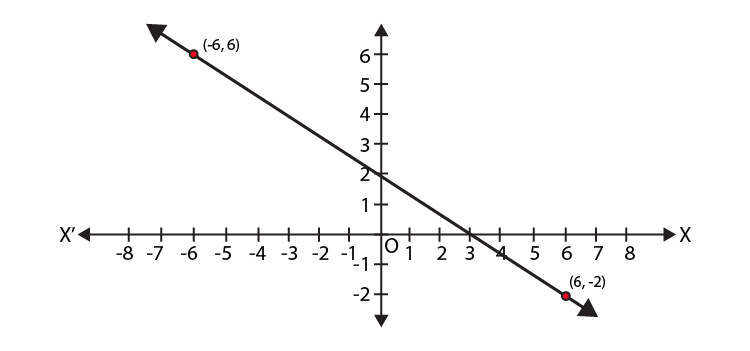We know that,

The linear equation of a line is,

y = mx + c, where, c is the y-intercept

From the graph,

We get y-intercept is 2.

⇒ c = 2.

Also, from the graph,

We get,

x1 = 6, y1 = – 2 and x2 = – 6, y2 = 6

We know that,

m = slope of the line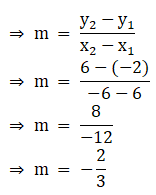∴ we get the linear equation,

y = – (2/3)x + 2

Multiplying whole equation by 3, we get,

⇒ 3y = – 2x + 6

⇒ 2x + 3y – 6 = 0

Thus, the points the graph of the linear equation cuts

(i)x-axis

Since, the point is on x axis, we have, y = 0.

Substituting y = 0 in the equation, 2x + 3y – 6 = 0,

We get,

2x + 3×0 – 6 = 0

⇒ 2x = 6

⇒ x = 3

Hence, the point at which the graph cuts x-axis = (3, 0).

(ii) y-axis

Since, the point is on y axis, we have, x = 0.

Substituting x = 0 in the equation, 2x + 3y – 6 = 0,

We get,

2×0 + 3y – 6 = 0

⇒ 3y = 6

⇒ y = 2

Hence, the point at which the graph cuts x-axis = (0, 2).

3. Draw the graph of the linear equation 3x + 4y = 6. At what points, the graph cuts X and Y-axis?

Solution:

According to the question,

We get the equation,

3x + 4y = 6.

We need at least 2 points on the graph to draw the graph of this equation,

Thus, the points the graph cuts

(i) x-axis

Since, the point is on x axis, we have, y = 0.

Substituting y = 0 in the equation, 3x + 4y = 6,

We get,

3x + 4×0 = 6

⇒ 3x = 6

⇒ x = 2

Hence, the point at which the graph cuts x-axis = (2, 0).

(ii) y-axis

Since, the point is on y axis, we have, x = 0.

Substituting x = 0 in the equation, 3x + 4y = 6,

We get,

3×0 + 4y = 6

⇒ 4y = 6

⇒ y = 6/4

⇒ y = 3/2

⇒ y = 1.5

Hence, the point at which the graph cuts x-axis = (0, 1.5).

Plotting the points (0, 1.5) and (2, 0) on the graph.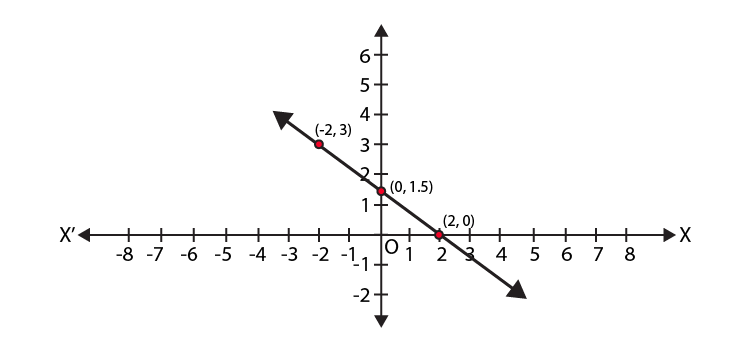BYJU’S have some more learning materials such as exemplar books, NCERT Solutions, notes, and questions papers, which students can use to prepare for the exam, thoroughly. Students are also recommended to solve sample papers and previous year question papers to know questions pattern asked from Maths Chapter 4 and marks contained by it.

## Frequently Asked Questions on NCERT Exemplar Solutions for Class 9 Maths Chapter 4

### List out the concepts covered in Chapter 4 of NCERT Exemplar Solutions for Class 9 Maths?

NCERT Exemplar Class 9 Maths Chapter 4 covers the topics which are given below:
Problems based on linear equations in two variables
Finding solutions to linear equations
Representation of linear equations in two variables, graphically
Linear equations of lines parallel to x-axis and y-axis in a graph

### Explain the concept of linear equations in two variables in Chapter 4 of NCERT Exemplar Solutions for Class 9 Maths.

Linear equations in two variables, explain the geometry of lines or the graph of two lines, plotted to solve the given equations. As we already know, the linear equation represents a straight line. The plotting of these graphs will help us to solve the equations, which consist of unknown variables. An equation is said to be a linear equation in two variables if it is written in the form of ax + by + c=0, where a, b & c are real numbers and the coefficients of x and y, i.e a and b respectively, are not equal to zero. Solutions for the exercise wise problems are also available in PDF format with the aim of helping students to score well in the annual exam.

### Why is the NCERT Exemplar Solutions for Class 9 Maths Chapter 4 PDF important from the exam point of view?

The NCERT Exemplar Solutions for Class 9 Maths Chapter 4 provides a clear idea about the Problems based on linear equations in two variables. By using the solutions PDF, students will get an in-depth knowledge about the principle of linear equations in two variables and its applications. These solutions will improve confidence among students to prepare for the Class 9 exams.